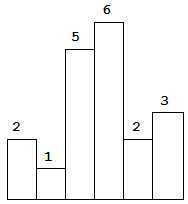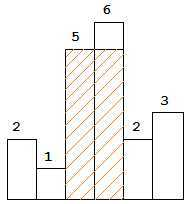# LeetCode: Largest Rectangle in Histogram

Given n non-negative integers representing the histogram’s bar height where the width of each bar is 1, find the area of largest rectangle in the histogram.Above is a histogram where width of each bar is 1, given height = `[2,1,5,6,2,3]`.The largest rectangle is shown in the shaded area, which has area = 10 unit.

For example,
Given height = `[2,1,5,6,2,3]`, return `10`.